# SQL Arithmetic Operators

## Arithmetic Operators

Arithmetic operators can perform arithmetical operations on numeric operands involved. Arithmetic operators are addition(+), subtraction(-), multiplication(*) and division(/). The + and - operators can also be used in date arithmetic.

Operator Meaning Operates on
- (Subtract) Subtraction Numeric value
* (Multiply) Multiplication Numeric value
/ (Divide) Division Numeric value
% (Modulo) Returns the integer remainder of a division. For example, 17 % 5 = 2 because the remainder of 17 divided by 5 is 2. Numeric value

Syntax:

```SELECT <Expression>[arithmetic operator]<expression>...
FROM [table_name]
WHERE [expression];
```
Parameter Description
Expression Expression made up of a single constant, variable, scalar function, or column name and can also be the pieces of a SQL query that compare values against other values or perform arithmetic calculations.
arithmetic operator Plus(+), minus(-), multiply(*), and divide(/).
table_name Name of the table.

Example: SQL Arithmetic Operators

This is a simple example of using SQL arithmetic operators:

``````SELECT 15+10-5*5/5 FROM dual;
``````

Relational Algebra Expression: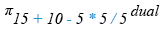Relational Algebra Tree: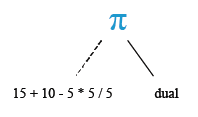## SQL plus (+) operator

The SQL plus (+) operator is used to add two or more expressions or numbers.

Example:

Sample table: customer

To get data of 'cust_name', 'opening_amt', 'receive_amt',  ('opening_amt' + 'receive_amt') from the 'customer' table with following condition -

1. sum of 'opening_amt' and  'receive_amt' is greater than 15000,

the following SQL statement can be used :

``````SELECT cust_name, opening_amt,
FROM customer
``````

Output:

```CUST_NAME                                OPENING_AMT RECEIVE_AMT (OPENING_AMT+RECEIVE_AMT)
---------------------------------------- ----------- ----------- -------------------------
Sasikant                                        7000       11000                     18000
Ramanathan                                      7000       11000                     18000
Avinash                                         7000       11000                     18000
Shilton                                        10000        7000                     17000
Rangarappa                                      8000       11000                     19000
Venkatpati                                      8000       11000                     19000
Sundariya                                       7000       11000                     18000
```

Relational Algebra Expression: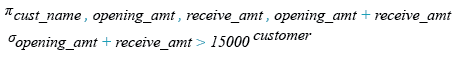Relational Algebra Tree: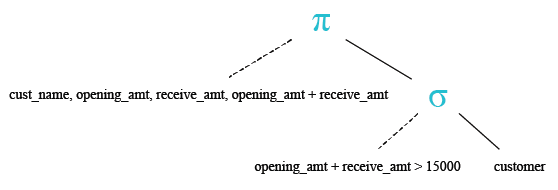## SQL minus (-) operator

The SQL minus (-) operator is used to subtract one expression or number from another expression or number.

Example:

To get data of 'cust_name', 'opening_amount', 'payment_amount' and 'oustanding_amount' from the 'customer' table with following condition -

1. 'outstanding_amt' - 'payment_amt' is equal to the  'receive_amt',

the following SQL statement can be used:/p>

``````SELECT cust_name,opening_amt, payment_amt, outstanding_amt
FROM customer
``````

Output:

```CUST_NAME                                OPENING_AMT PAYMENT_AMT OUTSTANDING_AMT
---------------------------------------- ----------- ----------- ---------------
Stuart                                          6000        3000           11000
```

Relational Algebra Expression: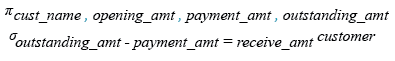Relational Algebra Tree: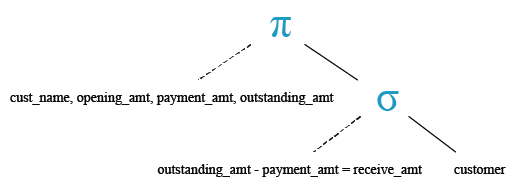## SQL multiply ( * ) operator

The SQL multiply ( * ) operator is used to multiply two or more expressions or numbers.

Example:

Sample table: agents

To get data of 'agent_code', 'agent_name', 'working_area' and  ('commission'*2) from the 'agents' table with following condition -

1. two times of the default 'commission' is greater than  0.25,

the following SQL statement can be used :

``````SELECT agent_code, agent_name,
working_area, (commission*2)
FROM agents
WHERE (commission*2)>0.25;
``````

Output:

```AGENT_ AGENT_NAME                               WORKING_AREA                        (COMMISSION*2)
------ ---------------------------------------- ----------------------------------- --------------
A003   Alex                                     London                                         .26
A001   Subbarao                                 Bangalore                                      .28
A007   Ramasundar                               Bangalore                                       .3
A011   Ravi Kumar                               Bangalore                                       .3
A010   Santakumar                               Chennai                                        .28
A005   Anderson                                 Brisban                                        .26
A006   McDen                                    London                                          .3
A004   Ivan                                     Torento                                         .3
```

Relational Algebra Expression: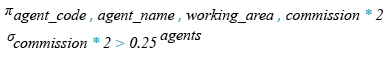Relational Algebra Tree: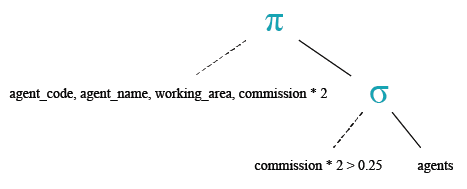## SQL divide ( / ) operator

The SQL divide ( / ) operator is used to divide one expressions or numbers by another.

Example:

To get data of 'cust_name',  'opening_amt',  'receive_amt',  'outstanding_amt' and ('receive_amt'*5/ 100) as a column heading  'commission' from the customer table with following condition -

1. 'outstanding_amt' is less than or equal to 4000,

the following SQL statement can be used :

``````SELECT cust_name, opening_amt, receive_amt,
FROM customer
WHERE outstanding_amt<=4000;
``````

Output:

```CUST_NAME                                OPENING_AMT RECEIVE_AMT OUTSTANDING_AMT COMMISSION
---------------------------------------- ----------- ----------- --------------- ----------
Holmes                                          6000        5000            4000        250
Bolt                                            5000        7000            3000        350
Karl                                            4000        6000            3000        300
Steven                                          5000        7000            3000        350
```

## SQL modulo ( % ) operator

The SQL MODULO operator returns the remainder (an integer) of the division.

Example:

To get the modulus of a division of 150 by 7 from the DUAL table, the following SQL statement can be used :

``````SELECT 150%7;
``````

Output:Practice SQL Exercises